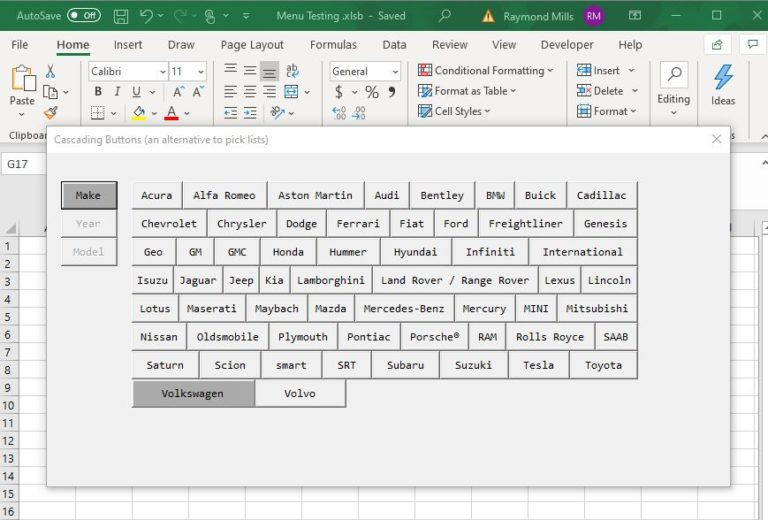We specialize in menu solutions that make what is at first blush complex – simple. Specifically how do you handle a large number of choices?   Here is some free code to get you thinking about the possibilities.VBA Cascading Menu buttons can be used as an alternative to cascading list  or combo boxes.   In some cases you simply wont have the vertical real estate to use either a combo boxes or list boxes.  In this example I use the Controls collection to build a very efficient cascading menu box.  Note: The 15,000 rows of source data is contained in an access database in the background –  Response time is instantaneous!
In this Brief Demo I take you through my vba cascading menu and show you how to use the Controls collection to make it easy.
```Private Sub UserForm_Initialize()

'****************************************************
'** Date:           10/08/2019
'** Developer:      Ray Mills
'**                 www.ExcelandVBACraftsman.com
'**                 with large number of choices
'**
'****************************************************

Dim strConn As String, sSql As String, sPath As String, sMisc As String
Dim i As Integer, w As Integer, p As Integer
DDim ileft As Integer, iLeftStart

'center the form
With Me
.StartUpPosition = 0
.Left = Application.Left + (0.5 * Application.Width) - (0.5 * .Width)
.Top = Application.Top + (0.5 * Application.Height) - (0.5 * .Height)
End With

'grab current path
sPath = Application.ActiveWorkbook.Path

' connect to accessdb ...
strConn = "Provider=Microsoft.ACE.OLEDB.12.0;" & _
Data Source= & sPath & "\Data for Menu Testing.accdb;" & _
Persist Security Info=False;
oConn.Open strConn

' Create a distinct model recordset ...
sSql = "SELECT DISTINCT Make FROM Auto_Database"

' set left and width ...
If btn1r.Width <> 48 Then
ileft = btn1r.Left: iLeftStart = btn1r.Left
Else
ileft = 72: iLeftStart = 72
End If

'loop thru the recordset & populate the button captions ...
i = 1: p = 1
Do Until oRs.EOF = True
sMisc = "btn" & i & "r"
Controls(sMisc).Width = w: Controls(sMisc).Left = ileft: ileft = ileft + w: p = p + 1
Controls(sMisc).Caption = oRs(0).Value: Controls(sMisc).Visible = False
oRs.MoveNext
i = i + 1

If p = 8 Then
ileft = iLeftStart
p = 1
End If
Loop

' deactivate year and model buttons until make is chosen ...
Me.btn2m.Enabled = False
Me.btn3m.Enabled = False

End Sub

```
Here is the essence of the code: Contols(“btn1r”).caption = oRs(0).value – In this case oRs(0).value = “Acura”. – Controls can be addressed by their name – Control properties can be changed by  Contols(control name).caption = “Alfa Romeo” Knowing this two tidbits should make my code easy to understand.
```Private Sub btn1m_MouseMove(ByVal Button As Integer, ByVal Shift As Integer, ByVal x As Single, ByVal Y As Single)

Dim myCntrl As Control
Dim strConn As String, sSql As String, sPath As String, sMisc As String
Dim i As Integer, w As Integer, p As Integer, ileft As Integer

'set the hover color, captions and enabled
btn1m.BackColor = &H8000000C: btn1m.Caption = "Make"
btn2m.BackColor = &H8000000F: btn2m.Caption = "Year": Me.btn2m.Enabled = False:
btn3m.BackColor = &H8000000F: btn3m.Caption = "Model": Me.btn3m.Enabled = False:

' clear the pic
Call Pic_Setup("999")

'grab current path
sPath = Application.ActiveWorkbook.Path

' connect to accessdb ...
strConn = "Provider=Microsoft.ACE.OLEDB.12.0;" & _
Data Source= & sPath & "\Data for Menu Testing.accdb;" & _
Persist Security Info=False;
oConn.Open strConn

sSql = "SELECT DISTINCT Make FROM Auto_Database "

i = 1: ileft = 72: p = 1
Do Until oRs.EOF = True
sMisc = "btn" & i & "r"
Controls(sMisc).Width = w: Controls(sMisc).Left = ileft: ileft = ileft + w: p = p + 1
Controls(sMisc).Caption = oRs(0).Value: Controls(sMisc).Visible = True
oRs.MoveNext
i = i + 1

If p = 9 Then
ileft = 72
p = 1
End If
Loop

' display the models
i = 1: ileft = 72: p = 1

' set the starting left & width ...
btn1m.Width = 48: btn2m.Width = 48: btn3m.Width = 48

Do Until oRs.EOF = True
sMisc = "btn" & i & "r"
Controls(sMisc).Width = w: Controls(sMisc).Left = ileft: ileft = ileft + w: p = p + 1
Controls(sMisc).Caption = oRs(0).Value: Controls(sMisc).Visible = False
oRs.MoveNext
i = i + 1

If p = 9 Then
ileft = 72
p = 1
End If
Loop
Call justify_tool

End Sub

```

If you were able to utilize what you learned here or improve the code,